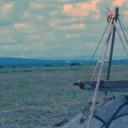# Raster attribute table to frequency table

483
3
06-30-2021 10:18 AM
Labels (2)by
New Contributor III

Is there a way to easy convert the attribute tables associated with a raster to a frequency table? I imagine that for most raster datasets such a table would be unwieldy

1 Solution

Accepted SolutionsbyMVP Esteemed Contributor

@DavidPike is correct.  A frequency table would have Value, Count as the fields... 2, 60

If the Value field were order and had values measured on an interval or ratio scale, the frequency table could be converted to an ogive (aka, cumulative frequency distribution) on of the fundamentals of statistics.

What you describe in an unravelled meshgrid.

... sort of retired...
3 RepliesbyMVP Frequent Contributor

Isn't the raster attribute table a frequency table?  I may be misunderstanding what a frequency table is, could you elaborate please.by
New Contributor III

Maybe I am misunderstanding. The attribute table groups cells with the same value. So, and you already know this, row one might have a value of 2 (whatever that is) with a cell count of, say, 60, meaning that 60 cells in the raster have a value of 2. Would not a frequency table have instead each cell listed as an individual in the table with a value of 2? This is not a huge issue since you ostensibly would not what the total number of cells are in the raster. But if you export the attribute table and treat it like a frequency table in some stats packages, it might assume the sample size is the number of rows, not the number of pixels.byMVP Esteemed Contributor

@DavidPike is correct.  A frequency table would have Value, Count as the fields... 2, 60

If the Value field were order and had values measured on an interval or ratio scale, the frequency table could be converted to an ogive (aka, cumulative frequency distribution) on of the fundamentals of statistics.

What you describe in an unravelled meshgrid.

... sort of retired...